Radius
Type
Sale/Rent
Min Price
Max Price
Min Beds
Max Beds
Advanced Options:
Advanced Search >

Close Advanced

# Property For Rent Showing properties 13 to 24 of 25

Sort by
• Let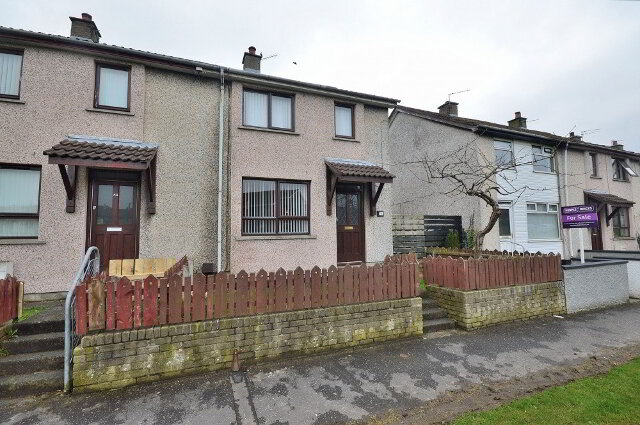LET
• Let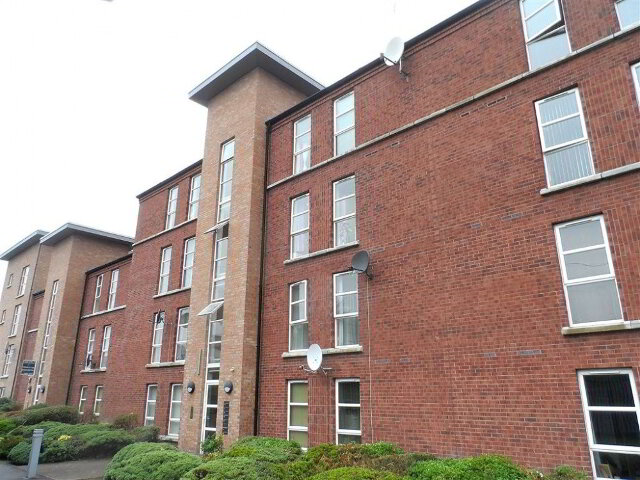LET
• LetLET
• Let agreedLet agreed £450 / month
• LetLET
• LetLET
• LetLET
• Let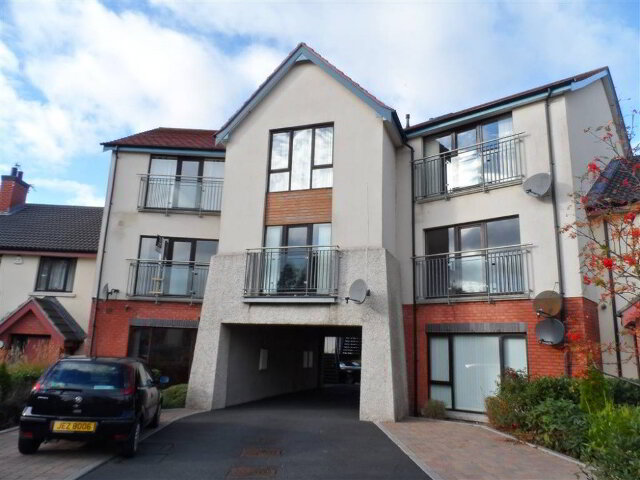LET
• Let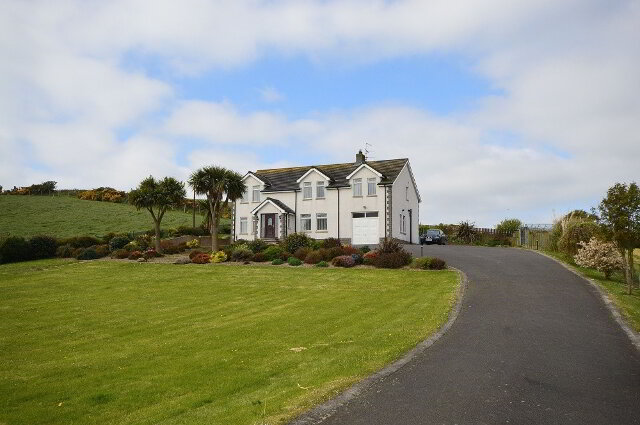LET
• Let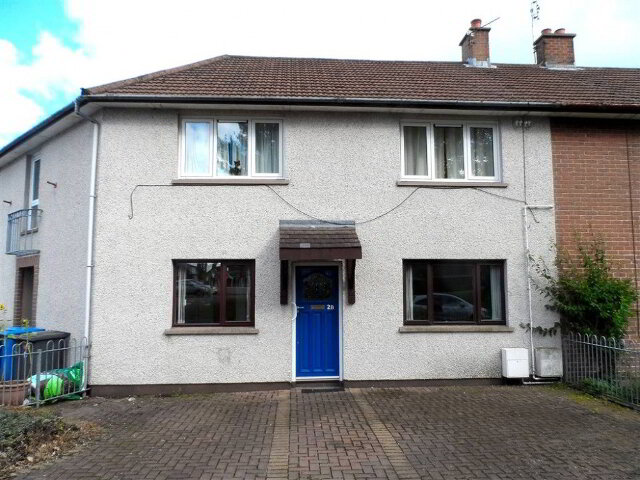LET
• Let agreedLet agreed £500 / month
• Let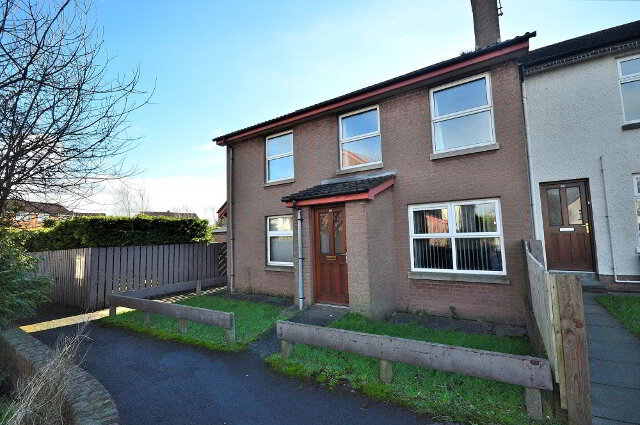LET